×#### Thank you for registering.

One of our academic counsellors will contact you within 1 working day.

Click to Chat

1800-1023-196

+91-120-4616500

CART 0

• 0

MY CART (5)

Use Coupon: CART20 and get 20% off on all online Study Material

ITEM
DETAILS
MRP
DISCOUNT
FINAL PRICE
Total Price: Rs.

There are no items in this cart.
Continue Shopping```Expressing Concentration of Solution

Table of Content

What is Concentration of Solution?

Methods of Expressing Concentration of Solutions

Percentage by Weight

Percentage by Volume

Weight by Volume

Mole Fraction

Parts Per Million

Molarity

Molality

Normality

Formality

Demal

What is Concentration of Solution?

The Concentration of a Solution is defined as the relative amount of solute present in a solution.

It basically talks about how to find the amount of solute present in solvent which together forms solution.There are various methods used to find this,

Methods of Expressing Concentration of Solutions

Percentage by weight (w / w %)

Percentage by volume (V / V%)

Weight by volume (w / v%)

Mole fraction (x)

Parts per million (ppm)

Molarity (M)

Molality (m)

Normality (N)

Formality (F)

Demal (D)

All of them are briefed below:

Percentage by Weight

Symbol: (w / w %)

Definition:  It is defined as the amount of solute present in 100 g of solution.

Unit:  No unit

Percentage by Volume

Symbol: (V / V %)

Definition:  It is defined as the volume of solute present in 100 mL of solution.

Unit:  No unit

Weight by Volume

Symbol: (W / V %)

Definition:  It is defined as the amount of solute present in 100 mL of solution.

Unit: mg/L

Formula:

Percent Concentration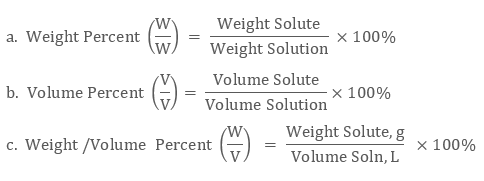Example:

As an example consider 5 g sugar dissolved in 20 g of water. What is the w/w% concentration of sugar in this solution?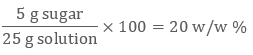Mole Fraction

Symbol :  X ( lower-case Greek letter chi, χ)

Definition:  It is the ratio of the number of moles of solute and the total number of moles of solute and solvent.

Unit: No unit

Formula: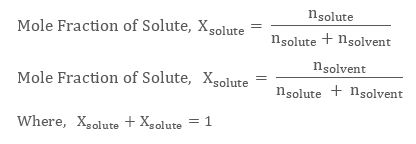Mole Fraction (X): This is the number of moles of a compound divided by the total number of moles of all chemical species in the solution.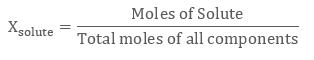Example:

What are the mole fraction of the components of the solution formed when 92 g glycerol is mixed with 90 g water? (molecular of weight water = 18; molecular weight of glycerol = 92)

Solution:

90 g water = 90 g x 1 mol / 18 g = 5 mol water

92 g glycerol = 92 g x 1 mol / 92 g = 1 mol glycerol

Total mol = 5 + 1 = 6 mol

X water = 5 mol / 6 mol = 0.833

X glycerol = 1 mol / 6 mol = 0.167

It’s a good idea to check your math by making sure the mole fractions add up to 1:

xwater + xglycerol = .833 + 0.167 = 1.000

Parts Per Million

Symbol:  ppm

Definition:  It is defined as the parts of a component per million parts (106) of the solution. It is widely used when a solute is present in trace quantities.

Unit: ppm

Formula: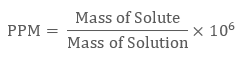Example:

Calculate the molar concentration of 2000 ppm of Pb2 + (A. M. = 207 g/mol)?

Solution:

PPM = M x M. M.x1000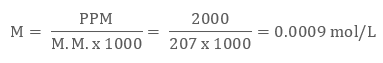Molarity:

Symbol :  M

Definition: Molarity of any solution is number of moles of solute per liter of solution

Unit: mol/litre

Formula:

Molarity = Number of moles of solute / Volume of solution in liter.Example:

What is the molarity of a solution containing 0.32 moles of NaCl in 3.4 liters?

Solution: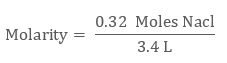= 0.94 M NaCl

Molality

Symbol:  m

Definition: Molality of any solution is represented as the number of moles of solute present per kg of solvent

Unit: mol/kg

Formula:

Molality(m) = Number of moles of solute / Mass of solvent in Kg

Note: Why m is not affected by change in temperature?

Since it is dependent on mass of solute and mass do not change to small shift in temperature due to close packing of solid atoms therefore it is independent of temperature change.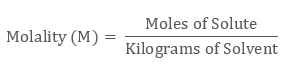Example:

What is the molarity of a solution containing 0.46 mole of solute in 2.0 kg water?

Given:

Moles of Solute = 0.46 mole

Kilogram of Solvent = 2.0 kgSolution:= 0.23 m

Normality

Symbol :  N

Definition: It is defined as the gram equivalent weight per liter of solution.

Unit: equivalent/L

Formula:

Normality = Number of grams – Equivalent of solute / Volume of solution in L

Number of gram-equivalents of solute = Mass of solute in gram / Equivalent weightExample:

Calculate the normality of 0.53 g/100 mL solution of Na2 CO3 (M.M. = 106 g/mol) as the following reaction:

Na2CO3 + 2H+ → H2CO3 + 2Na

Solution:

Eq. wt. of Na2 CO3 = 106/2 = 53 g/eq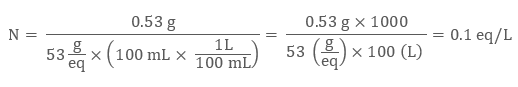Formality

Symbol :  F

Definition: It is the number of formula weights of solute present per liter of the solution.

Unit:  mol/L

Formula: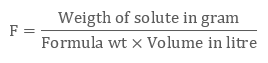It is applicable in case of ionic solids like NaCI.

Example:

Calculate the formality of solution in which 948 gram potash alum is dissolved in 5 litre solution.

Solution:

The formula mass of potash alum is 474 gram (formula mass)-1.Demal

Symbol:  D

Definition: It represents one mole of solute present in 1L of solution at O°C.

Watch this Video for more reference

```### Course Features

• 731 Video Lectures
• Revision Notes
• Previous Year Papers
• Mind Map
• Study Planner
• NCERT Solutions
• Discussion Forum
• Test paper with Video Solution